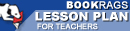Lesson Plans

# The Logic of Scientific Discovery Test | Final Test - Easy

This set of Lesson Plans consists of approximately 102 pages of tests, essay questions, lessons, and other teaching materials.
 View a FREE sampleName: _________________________ Period: ___________________

This test consists of 15 multiple choice questions and 5 short answer questions.

## Multiple Choice Questions

1. What is Popper's goal in researching a theory?
(a) To determine its value.
(b) To determine if it is a singular statement.
(c) To determine if it is probable.
(d) To determine if it is corroborated.

2. What type of numerical interpretation requires that probabilities be only expressed as frequencies?
(a) Non-empirical.
(b) Subjective.
(c) Psychological.
(d) Objective.

3. What does Popper feel about an axiom in relations of von Mises view of an axiom of randomness?
(a) It is a weak construct.
(c) It is a powerful construct.
(d) Mathematical validity is high.

4. What can be predicted using the statistical scatter principle?
(a) A particle's path.
(b) A particle's mass.
(c) A particle's speed.
(d) A particle's size.

5. What is used to make laws based on observations?
(a) Theory.
(b) Simplicity.
(c) Conventionalism.
(d) Probability.

6. How do conventionalists define simplicity?
(a) As a unified practice.
(b) Unpractical.
(c) Aesthetic and practical.
(d) As a means to an end.

7. What relationships does Popper explore in chapter 8?
(a) Probability and experience.
(b) Probability and assumptions.
(c) Probability and simplicity.
(d) Probability and scientific fact.

8. What is directly linked to falsification?
(a) Theory.
(b) Statements.
(c) Testability.
(d) Logic.

9. Who does Popper suggest works in probabilistic logic to determine the probability of a hypothesis?
(a) Inductivists.
(b) Positivist.
(c) Conventionalists.
(d) Metaphysicists.

10. What theory attempted to eliminate unobservable events?
(a) Einstein's theory of probability.
(b) Heisenberg's uncertainty principle.
(c) The Bohr Model.
(d) Newton's theory of gravity.

11. If a system is protected what will its degree of falsifiability be equal to?
(a) 1.
(b) -1.
(c) 0.
(d) 2.

12. What is the most significant factor when selecting a theory?
(a) Previous research.
(b) Its universality.
(c) The degree of testability.
(d) The understandability.

13. How does Popper explain the differences in his selection process?
(a) Using ordinal numbers.
(b) Using rational numbers.
(c) Using discrete categories.
(d) Using letters.

14. What involves considering the number of favorable cases divided by the number of possible cases?
(a) Abstract probability.
(b) Numerical probability.
(c) Non-numerical probability.
(d) Concrete probability.

15. What scientific thought suggests that probability can be regarded as a generalization of the concept of a singular truth?
(a) Naturalism.
(b) Conventionalism.
(c) Inductivism.
(d) Positivism.

1. What theory does Heisenberg begin this investigation with?

2. What does Popper eliminate from the common frequency theory by using mathematical and narrative approaches?

3. What does Popper assume is a main characteristic of quantum theory formula?

4. Why does Popper find Heisenberg's lack of logic and statistical testing disturbing?

5. What principle does science presuppose?

 This section contains 437 words (approx. 2 pages at 300 words per page) View a FREE sample